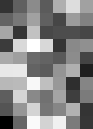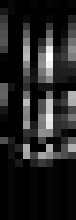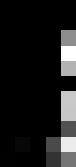## An Intro to Convolutional Networks in Torch

This tutorial will focus on giving you working knowledge to implement and test a convolutional neural network with torch. If you have not yet setup your machine, please go back and give this a read before starting.

You can start iTorch by opening a console, navigating to <install dir>/torch/iTorch and typing ‘itorch notebook’. Once this is done, a webpage should pop up, which is served from your own local machine. Go ahead and click the ‘New Notebook’ button.

Because we’re just starting out, we will start with a very simple problem to solve. Suppose you have a signal which needs to be classified as either a square pulse, or a triangular pulse. Each pulse is sampled over time. To make the problem slightly more challenging, lets say the pulse is not always in the same place, and the pulse can have constrained but random height and width. There are several techniques we could use to solve this problem. We could do signal processing such as taking the FFT, or we could code up our own custom filters. But that involves work, and also becomes impossible when faced with larger problems. So what do we do? We can build a convolutional neural network!

Convolutional Networks
Convolutional Layers
The network will start out with a 64×1 vector, which we can effectively call a 1-D vector with each value representing the signal strength at each point in time. Next we apply a convolution of those 64 points with ten kernels, each with 7-elements. These kernel weights will act as filters, or features. We don’t know yet what the values will be, since they will be learned as we train the network. Layers of the network that take an input, and convolve on ore more filters to create an output are called convolutional layers. Example:

Convolution 3×1 kernel, 8×1 input

Input: 2 4 3 6 5 3 7 6
Kernel values: -1 2 -1
Output: 3 -4 4 1 -6 5
Further explanation: (-1*2)+(2*4)+(-1*3) = 3

Pooling layer
After convolutional layers, there is frequently a pooling layer. This layer is used to reduce the problem size, and thus speed up training greatly. Typically, MaxPooling is used, which acts like a king of convolution, except that it has a stride usually equal to the kernel size, and the ‘kernel’ really just takes the maximum value of the input, and outputs that maximum value. This is great for classification problems such as this, because the position of the signal isn’t very important, just whether it is square or triangular. So pooling layers throw away some positioning data, but make the problem smaller and easier to train. Example:

Max pooling layer, size 2, stride 2
Input: 3 5 7 6 3 4
Output: 5 7 4
Further explanation: Max(3,5) = 5, Max(7,6) = 7, Max(3,4) = 4

Activation Function
Neural networks achieve their power by introducing non-linearities into the system. Otherwise, networks just become big linear algebra problems, and there is no point in having many layers. In days past, the sigmoid used to be most common, however, recent breakthroughs have indicated that ReLU is a much better operator for deep neural networks. Basically, it is just ‘y = max(0,x)’. So if x is negative, y is 0, otherwise, y is equal to x. Example:

Input: 4 6 2 -4
Output: 4 6 2 0

That is enough talking, time for doing! Go ahead and launch an itorch notebook.

First things first, be sure to include the neural network package.

```-- First, be sure to require the 'nn' package for the neural network functions
require 'nn';```

Next, we’ll need to create some training data. Neural networks require many examples in order to train, so we choose to generate 10000 example signals. This number may seem large, but remember that we have 4 randomized components to each wave; Type, height, width, start index. This translates to 2*6*21*6 = 1512 possible permutations. In real life, problems are much more complex.

```-- Next, create the training data. We'll use 10000 samples for now
nExamples = 10000

trainset = {}
trainset.data = torch.Tensor(nExamples,64,1):zero() -- Data will be sized as 5000x64x1
trainset.label = torch.Tensor(nExamples):zero()     -- Use one dimensional tensor for label

--The network trainer expects an index metatable
setmetatable(trainset,
{__index = function(t, i)
return {t.data[i], t.label[i]}  -- The trainer is expecting trainset to be {data, label}
end}
);

--The network trainer expects a size function
function trainset:size()
return self.data:size(1)
end

function GenerateTrainingSet()

-- Time to prepare the training set with data
-- At random, have data be either a triangular pulse, or a rectangular pulse
-- Have randomness as to when the signal starts, ends, and how high it is
for i=1,nExamples do
curWaveType = math.random(1,2)      -- 1 for triangular signal, 2 for square pulse
curWaveHeight = math.random(5,10)   -- how high is signal
curWaveWidth = math.random(20,40)   -- how wide is signal
curWaveStart = math.random(5,10)    -- when to start signal

for j=1,curWaveStart-1 do
trainset.data[i][j] = 0
end

if curWaveType==1 then   -- We are making a triangular wave
delta = curWaveHeight / (curWaveWidth/2);
for curIndex=1,curWaveWidth/2 do
trainset.data[i][curWaveStart-1+curIndex] = delta * curIndex
end
for curIndex=(curWaveWidth/2)+1, curWaveWidth do
trainset.data[i][curWaveStart-1+curIndex] = delta * (curWaveWidth-curIndex)
end
trainset.label[i] = 1
else
for j=1,curWaveWidth do
trainset.data[i][curWaveStart-1+j] = curWaveHeight
end
trainset.label[i] = 2
end
end
end

GenerateTrainingSet()
```

Next, we will construct our neural network. Starting with 64×1 data going in, we will go two Convolution-MaxPool-ReLU ‘layers’, and end with a two layer fully connected neural network, and end with two outputs. Because this is a classification problem, we’ll use log-probability output. Whichever output is greatest (close to zero) is the selection of the network. The other output should have a negative value.

```-- This is where we build the model
model = nn.Sequential()                       -- Create network

-- First convolution, using ten, 7-element kernels
model:add(nn.TemporalConvolution(1, 10, 7))   -- 64x1 goes in, 58x10 goes out
model:add(nn.TemporalMaxPooling(2))           -- 58x10 goes in, 29x10 goes out

-- Second convolution, using 5, 7-element kernels
model:add(nn.TemporalConvolution(10, 5, 7))   -- 29x10 goes in, 23x5 goes out
model:add(nn.TemporalMaxPooling(2))           -- 23x5 goes in, 11x5 goes out

-- After convolutional layers, time to do fully connected network
model:add(nn.View(11*5))                        -- Reshape network into 1D tensor

model:add(nn.Linear(11*5, 30))                  -- Fully connected layer, 55 inputs, 30 outputs

model:add(nn.Linear(30, 2))                     -- Final layer has 2 outputs. One for triangle wave, one for square
model:add(nn.LogSoftMax())                      -- log-probability output, since this is a classification problem```

With torch, we can see the dimensions of a tensor by applying a ‘#’ before it. So at any time when constructing the network, you can create a partially complete network, and propagate a blank tensor through it and see what the dimension of the last layer is.

```-- When building the network, we can test the shape of the output by sending in a dummy tensor
#model:forward(torch.Tensor(64,1))```

Next, we set our criteria to nn.ClassNLLCriterion, which is helpful for classification problems. Next, we create a trainer using the StochasticGradient descent algorithm, and set the learning rate and number of iterations. If the learning rate is too high, the network will not converge. If it is too low, the network will converge too slowly. So it takes practice to get this just right.

```criterion = nn.ClassNLLCriterion()
trainer.learningRate = 0.01
trainer.maxIteration = 200 -- do 200 epochs of training
```

Finally, we train our model! Go grab a cup of coffee, it may take a while. Later we will focus on accelerating these training sessions with the GPU, but our network is so small right now that it isn’t practical to accelerate.

`trainer:train(trainset)`

We can see what an example output and label are below.

```-- Lets see an example output
model:forward(trainset.data)

-- Lets see which label that is
trainset.label```

Let’s figure out how many of the examples are predicted correctly.

```function TestTrainset()
correct = 0
for i=1,nExamples do
local groundtruth = trainset.label[i]
local prediction = model:forward(trainset.data[i])
local confidences, indices = torch.sort(prediction, true)  -- sort in descending order
if groundtruth == indices then
correct = correct + 1
else
--print("Incorrect! "..tostring(i))
end
end
print(tostring(correct))
end

-- Lets see how many out of the 10000 samples we predict correctly!
TestTrainset()```

Hopefully, that number should read 10,000. Next, let’s be sure our network is really trained well. Let us generate new training sets, and test them. Hopefully, everything will be 10,000, but if there are some incorrect examples, go back and train some more. In real life, we can suffer from a phenomenon called over-training where the model is over-fit to our training data, but we will cover this in a later article. Try to train your network until it passes everything you can throw at it.

```-- Generate a new set of data, and test it
for i=1,10 do
GenerateTrainingSet()
TestTrainset()
end```

Great, you’ve done it! Now, lets try to gain some understanding into what’s going on here. We created two convolutional layers, the first having ten 1×7 kernels, and the second convolutional layer having five, 10×7 kernels. The reason I use itorch instead of the command line torch interface is so I can easily inspect graphics. Let’s take a look at the filter in the first convolutional layer. We can see that each row is a filter.

```require 'image'
itorch.image(model.modules.weight)```We can also see which neurons activate the most. You can propagate any input through the network with the :forward function, as demonstrated earlier. Then, we can visualize the outputs of the ReLU (or any) layers. For example, here is the output of the first ReLu layer. It is obvious that some filters are activating more than others.

`itorch.image(model.modules.output)`Next, lets take a look at the next ReLu layer output. Here we can see that the neurons in the 5th layer are by far the most active for this input. So we know that even if our filters look a little chaotic, neurons in a particular layer do activate and stand out. Finally, these values are sent to the fully connected neural network, which makes sense of what it means when different filters are activated in relation to other filters.

`itorch.image(model.modules.output)`Now that we understand how different filters activate with certain inputs, let us introduce noise into system and see how the neural network deals with this.

```function IntroduceNoise()
for i=1,nExamples do
for j=1,64 do
trainset.data[i][j] = trainset.data[i][j] + torch.normal(0,.25);
end
end
end

-- Generate a new set of data, and test it
for i=1,10 do
GenerateTrainingSet()
IntroduceNoise()
TestTrainset()
end```

After training my network around 600 epochs, I was able to achieve 100% perfect signal categorization with the noisy inputs, even though I only trained on the noiseless inputs. Wow! This shows us that the network does indeed work, and is powerful enough to filter out noise which happens in real life data. Next, we will be ready for more interesting challenges!

```-- To see the network's structure and variables
model.modules
```